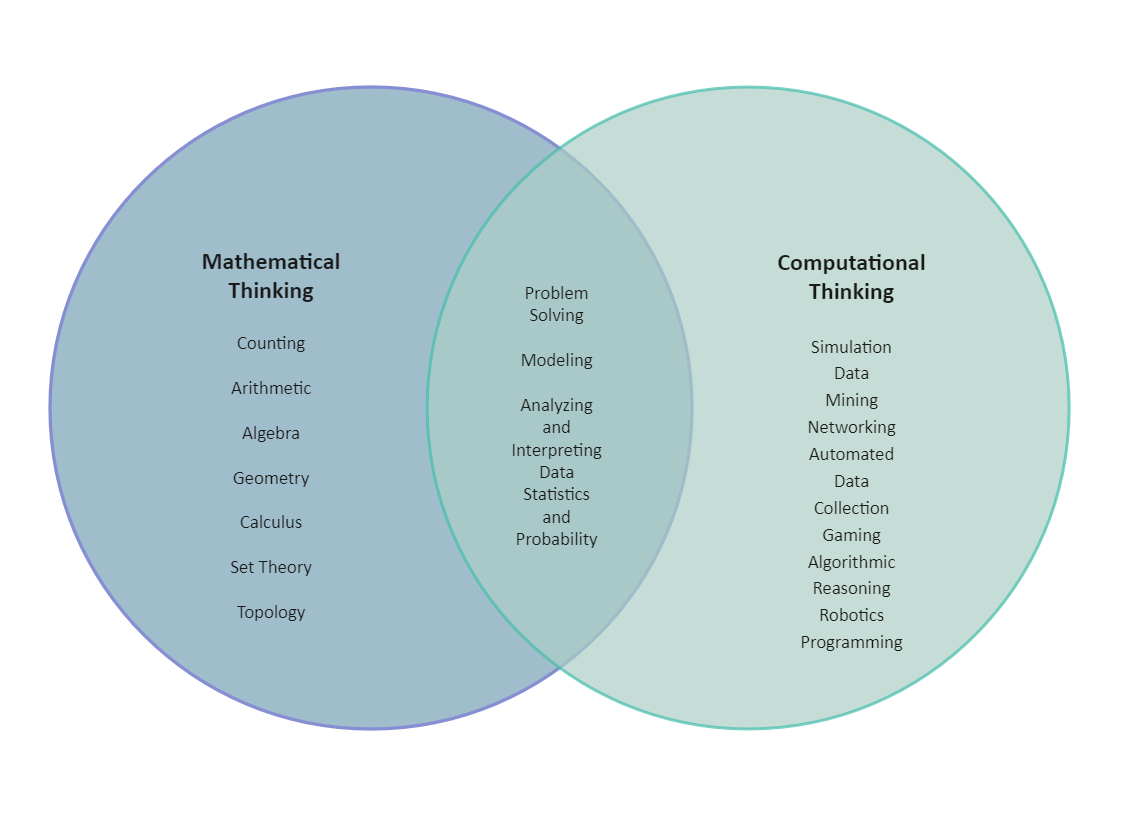Template Community / Venn Diagram Math

# Venn Diagram MathBlackwood
Published on 2022-04-20
Edit OnlineA Venn Diagram Math is a graphical depiction of the similarities and differences between two or more concepts. It uses circles to illustrate relationships between two or more sets of data. Each circle represents a different set of data. A Venn Diagram Math is used in mathematics to visualize the logical relationship between sets and their elements and to help us solve examples based on these sets. A Venn diagram typically uses intersecting and non-intersecting circles (although other closed figures such as squares may be used). When we use a set, it is easier to start with a larger set called a universal set, which contains all of the elements in all of the sets under consideration.
Tag
venn diagram
Venn Diagram Collection
2 Set Venn Diagram
Report
1
89PostRecommended Templates